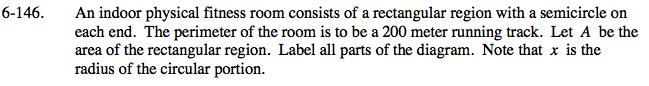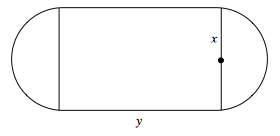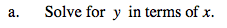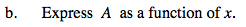### Home > PC > Chapter 6 > Lesson 6.4.1 > Problem6-146

6-146.
1. An indoor physical fitness room consists of a rectangular region with a semicircle on each end. The perimeter of the room is to be a 200 meter running track. Let A be the area of the rectangular region. Label all parts of the diagram. Note that x is the radius of the circular portion. Homework Help ✎1. Solve for y in terms of x.

2. Express A as a function of x.Circumference = 2πr (or 2πx in this case)

200 = 2y + 2πxUse the information from part (a).

A = xy + πx2